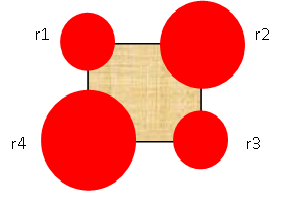# Practice on Toph

Participate in exhilarating programming contests, solve unique algorithm and data structure challenges and be a part of an awesome community.

# Around the Square

By battirunner · Limits 1s, 512 MB

You are given a square and there are four circles which are overlapping the square. The center of each four circles is one of the four vertices of that square. The radii of these circles are given in a way so that none of the circles overlap with each other but may touch.You are given a simple task to calculate the part of the area of the square which is not covered by the circles.

## Input

In each line, there are five numbers a, r1, r2, r3, r4 where a represents the length of the sides of the square and r1, r2, r3, r4 represent the radii of the 1st, 2nd , 3rd and 4th circles respectively as shown in the figure.

You can assume that all of these numbers are positive and fit into a 32 bit integer.

Input will be terminated at end-of-file (EOF).

## Output

For each test case print the area of the square not covered by any of the circles. Print the area to 3 decimal points.

## Sample

InputOutput
10 5 5 5 5
8 3 2 3 2
5 0 1 2 3
21.460
43.580
14.004

Use acos(-1) as the value of π (pi).GeometryuDebug

### Statistics

87% Solution Ratio

mjtbasifEarliest, Jun '16

Baka_RaffiFastest, 0.0s

Fazlerabbi.Lightest, 0 B

joe.masterShortest, 111B

### Submit

 EWU Individual Programming Battle Summer 2016Jun '16 AUST CSE 44th Batch Practise Contest 04Apr '20